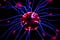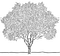# Does padding matter in deep learning models?

The article presents two experiments that show the influence of padding in deep learning models.

# Experiment 1

Convolution is shift-equivariant: shifting the input image by 1 pixel shifts the output image also by 1 pixel (see Fig. 1). If we apply global average pooling to the output (i.e., sum over all pixel values), we get a shift-invariant model: no matter how we shift the input image, the output will stay the same. In the language of PyTorch the model looks like this: y = torch.sum(conv(x), dim=(2, 3)) with input x and output y.

# What a text recognition system actually sees

## Some insights into the neural network “black box” of a text recognition systemLet’s have a look at what happens inside the neural network “black box” of a text recognition system.

The performance of modern text recognition systems implemented as neural networks is amazing. They can be trained on medieval documents and are able to read them and only make very few mistakes. Such tasks would be very difficult for most of us: look at Fig. 1 and give it a try!

# GPU Image Processing using OpenCL

## Implementation of two image processing methods in less than 120 lines of code using Python and OpenCL

Besides the obvious use-case of a Graphics Processing Unit (GPU), namely rendering 3D objects, it is also possible to perform general-purpose computations using frameworks like OpenCL or CUDA. One famous use-case is bitcoin mining. We will look at an other interesting use-case: image processing. After discussing the basics of GPU programming, we implement dilation and erosion in less than 120 lines of code using Python and OpenCL.

# Why is image processing well suited for GPUs?

## First reason

Many image processing operations iterate from pixel to pixel in the image, do some calculation using the current pixel value, and finally write each computed value to an output image. Fig. 1 shows…

# FAQ: Build a Handwritten Text Recognition System using TensorFlow

There were some questions which I want to discuss here. Let’s have a look at the following three ones:

1. How to recognize text in your images/datasets?
2. How to recognize text contained in lines or full pages?
3. How to compute a confidence score for the recognized text?

# 1 How to recognize text in your images/datasets?

The pre-trained model was trained on the IAM-dataset. One sample from IAM is shown in Fig. 1. The model not only learns how to…

# Word Beam Search: A CTC Decoding Algorithm

## Improve text recognition results: avoid spelling mistakes, allow arbitrary numbers and punctuation marks and make use of a word-level language modelTrees are an essential ingredient of the presented algorithm. (Taken from http://turnoff.us/geek/binary-tree with changes)

Let’s suppose we have a neural network (NN) for text recognition (OCR or HTR) which is trained with the connectionist temporal classification (CTC) loss function. We feed an image containing text into the NN and get the digitized text out of it. The NN simply outputs the characters it sees in the image. This purely optical model might introduce errors because of similar looking characters like “a” and “o”.

Problems like the one shown in Fig. 1 will look familiar to you if you have already worked on text-recognition: the result is of course wrong, however, when we look closely…

# Beam Search Decoding in CTC-trained Neural Networks

## A fast and well-performing algorithm with integrated language model to decode the neural network output in the context of text recognition

Neural networks (NN) consisting of convolutional NN layers and recurrent NN layers combined with a final connectionist temporal classification (CTC) layer are a good choice for (handwritten) text recognition.

The output of the NN is a matrix containing character-probabilities for each time-step (horizontal position), an example is shown in Fig 1. This matrix must be decoded to get the final text. One algorithm to achieve this is beam search decoding which can easily integrate a character-level language model.

# Build a Handwritten Text Recognition System using TensorFlow

## A minimalistic neural network implementation which can be trained on the CPU

Offline Handwritten Text Recognition (HTR) systems transcribe text contained in scanned images into digital text, an example is shown in Fig. 1. We will build a Neural Network (NN) which is trained on word-images from the IAM dataset. As the input layer (and therefore also all the other layers) can be kept small for word-images, NN-training is feasible on the CPU (of course, a GPU would be better). This implementation is the bare minimum that is needed for HTR using TF.

# An Intuitive Explanation of Connectionist Temporal Classification

## Text recognition with the Connectionist Temporal Classification (CTC) loss and decoding operation

If you want a computer to recognize text, neural networks (NN) are a good choice as they outperform all other approaches at the moment. The NN for such use-cases usually consists of convolutional layers (CNN) to extract a sequence of features and recurrent layers (RNN) to propagate information through this sequence. It outputs character-scores for each sequence-element, which simply is represented by a matrix. Now, there are two things we want to do with this matrix:

1. train: calculate the loss value to train the NN
2. infer: decode the matrix to get the text contained in the input image